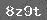# 第四单元达标自测题

（一）填空题。

118÷3= 6，被除数是（    ），除数是（    ），商是（    ），读作（    ）除以（    ）等于（    ）。

12÷4= 3，表示把（    ）平均分成（    ）份，每份是（    ），读作（    ）除以（    ）　等于（    ）。

2●●●●●●8÷2

8里面有（    ）个（    ）。

3．根据图意填空并写出算式。①表示把（    ）个平均分成（    ）份，每份（    ）个。

②（    ）里面有（    ）个（    ）。

③算式：□○□=□○□=

（二）在□里填上“＋”“- “×”、“÷”

5 6= 30    4 6= 24   4 4= 1

8 12= 20   9 9= 0   12 3= 4

18 12= 30  186=3   8 4=2

（三）在□里填上“> ”、“< ”或“= ”。

10÷25    1×3 8÷2    25÷54

4 4÷4     20 4×5       13 3×5

2×222  5×210       4163×4

4 6÷

  下一页

### 相关推荐

 留言与评论（共有 0 条评论）

 验证码：